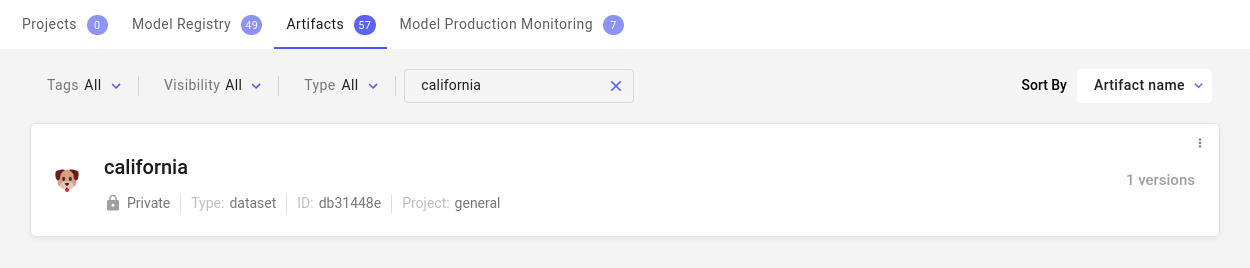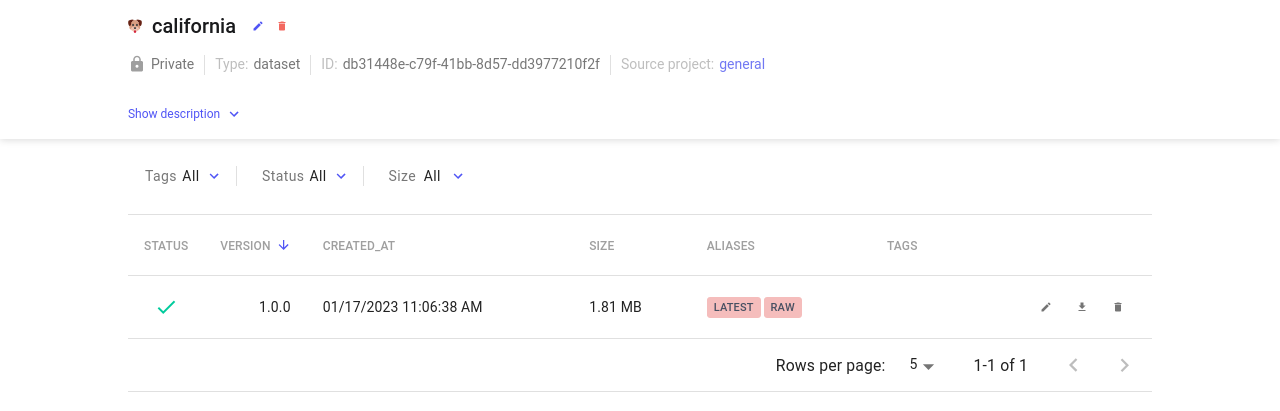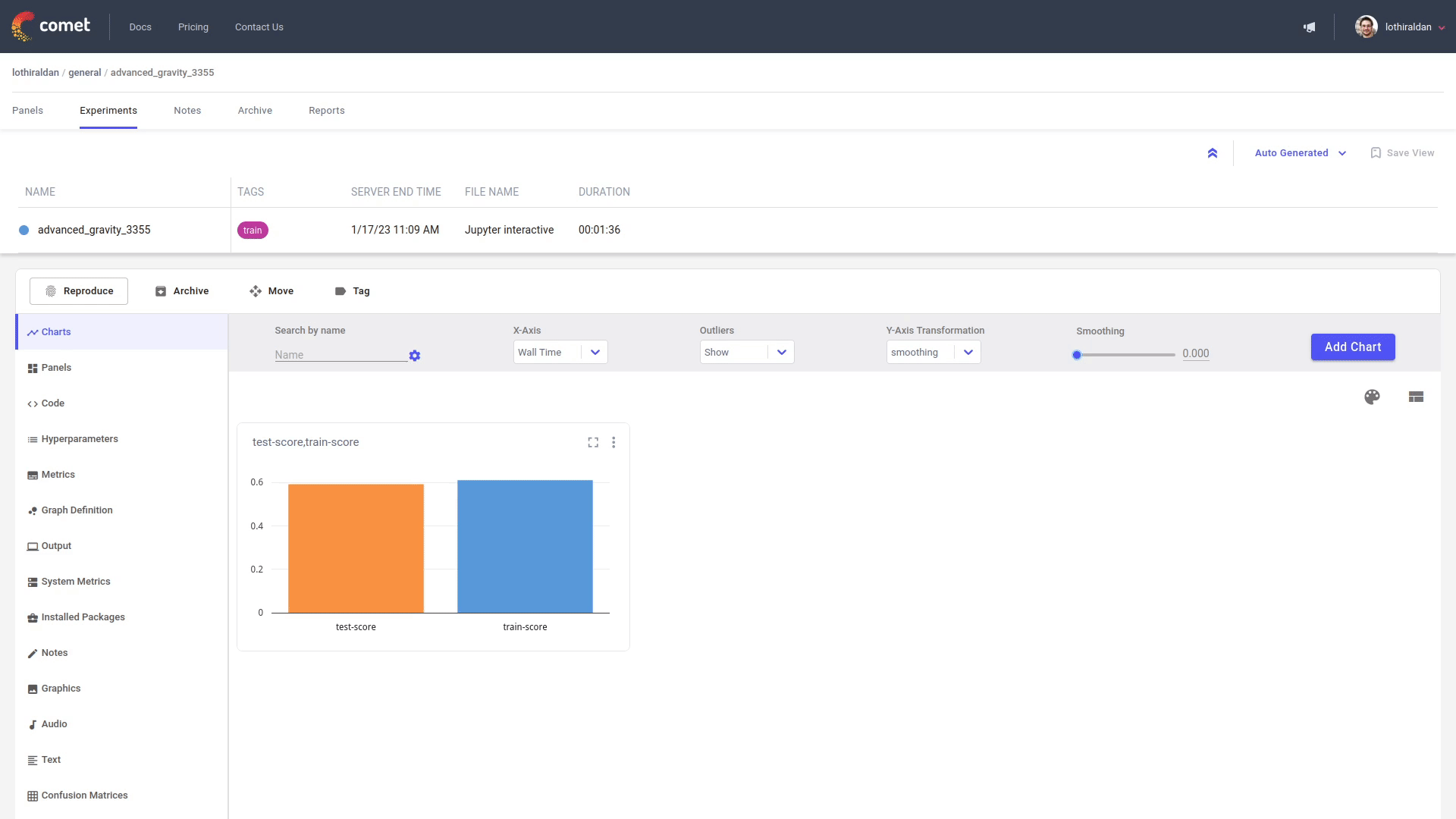# Example: Create and use Artifacts with your Experiments¶

This hands-on examples takes you through the following steps:

1. First, you'll download the California Housing Prices dataset, and save it to Comet as an Artifact.
2. You'll use this Artifact to train a simple regression model and log the trained model to Comet as an Artifact.
3. Then, you'll see how an Experiment tracks the input and output Artifacts produced in this process.
4. Next, you'll apply some preprocessing to your data, update your dataset Artifact and retrain the model to see if this improves your training metrics.

## Create an Artifact¶

Start by fetching the data.

``````import os
import pandas as pd
from sklearn.datasets import fetch_california_housing as load_data
from sklearn.model_selection import train_test_split

X, y = dataset.data, dataset.target
featurecols = dataset.feature_names

# Train-Test Split
X_train, X_test, y_train, y_test = train_test_split(X, y, random_state=42)

train_df = pd.DataFrame(X_train, columns=featurecols)
test_df = pd.DataFrame(X_test, columns=featurecols)

train_df["target"] = y_train
test_df["target"] = y_test

os.makedirs("./datasets", exist_ok=True)

train_df.to_csv("./datasets/train.csv", index=False)
test_df.to_csv("./datasets/test.csv", index=False)
``````

``````import comet_ml

# Create a Comet Artifact
artifact = comet_ml.Artifact(
name="california",
artifact_type="dataset",
aliases=["raw"],
)

# Add files to the Artifact
for split, asset in zip(
["train", "test"], ["./datasets/train.csv", "./datasets/test.csv"]
):

experiment = comet_ml.Experiment(
)
experiment.log_artifact(artifact)

experiment.end()
``````

Uploaded Artifacts are displayed at the Workspace level and are accessible across your Projects.Clicking on the Artifact brings up the information such as the date it was created, description, existing versions, and aliases.Note

There are alternatives to setting the API key programatically. See more here.

## Use the Artifact¶

Now that you've set up your Artifact, use it to train a linear regression model.

``````experiment = comet_ml.Experiment(
)

# Fetch the Artifact object from Comet
name = 'california'
version_or_alias = 'raw'
artifact = experiment.get_artifact(name, version_or_alias=version_or_alias)

output_path = "./artifacts"
``````

### Train a Model¶

``````from sklearn.linear_model import LinearRegression
from joblib import dump

y_train = train_df.pop("target").values
X_train = train_df.values

y_test = test_df.pop("target").values
X_test = test_df.values

# Initialize Model
model = LinearRegression()
model.fit(X_train, y_train)

# Evaluate Model
train_score = model.score(X_train, y_train)
test_score = model.score(X_test, y_test)

experiment.log_metric("train-score", train_score)
experiment.log_metric("test-score", test_score)

# Save Model
model_path = "./linear-model.pkl"
dump(model, model_path)
``````

### Log the Model as an Artifact¶

Once you've finished training, save the model binary as an Artifact so that you can re-use the model later on.

``````# Log Model as an Artifact
model_artifact = comet_ml.Artifact(
"housing-model", artifact_type="model", aliases=["baseline"]
)
experiment.log_artifact(model_artifact)
``````

You can view the Artifacts produced and consumed by an Experiment in the Assets and Artifacts tab under Artifacts. Toggle the direction selector to filter by Input, which refers to Artifacts that were consumed, and Output which refers to Artifacts that were produced.## Update the Artifact¶

You just trained a model on the unprocessed dataset. Now, apply some scaling to the input features, update the dataset Artifact, and rerun the training.

### Apply standard scaling to the dataset¶

``````from sklearn.preprocessing import StandardScaler as Scaler

X_scaler = Scaler().fit(X_train)
y_scaler = Scaler().fit(y_train.reshape(-1, 1))

X_train_scaled = X_scaler.transform(X_train)
X_test_scaled = X_scaler.transform(X_test)

y_train_scaled = y_scaler.transform(y_train.reshape(-1, 1))
y_test_scaled = y_scaler.transform(y_test.reshape(-1, 1))

train_scaled_df = pd.DataFrame(X_train, columns=featurecols)
test_scaled_df = pd.DataFrame(X_test, columns=featurecols)

train_scaled_df["target"] = y_train
test_scaled_df["target"] = y_test

train_scaled_df.to_csv("./datasets/train-scaled.csv")
test_scaled_df.to_csv("./datasets/test-scaled.csv")
``````

### Update the dataset Artifact¶

When updating the Artifact, notice how the alias `standard-scaled`has been added. This will make it easier to refer to this Artifact when you fetch it in the future.

``````import comet_ml

experiment = comet_ml.Experiment(
)

# Update Artifact with Scaled Data
scaled_dataset_artifact = comet_ml.Artifact(
"california",
artifact_type="dataset",
aliases=["standard-scaled"],
)

# Add files to the Artifact
for split, asset in zip(
["train", "test"], ["./datasets/train-scaled.csv", "./datasets/test-scaled.csv"]
):
)

experiment.log_artifact(scaled_dataset_artifact)
experiment.end()
``````

Note

There are alternatives to setting the API key programatically. See more here.

### Train a Model on a new Artifact¶

Notice in this snippet, that the code to fetch the Artifact is the same, with the exception of the alias.

``````experiment = comet_ml.Experiment(
)

# Fetch the Artifact object from Comet
name = 'california'
version_or_alias = 'standard-scaled'
artifact = experiment.get_artifact(name, version_or_alias=version_or_alias)

output_path = "./artifacts"

y_train = train_df.pop('target').values
X_train = train_df.values

y_test = test_df.pop('target').values
X_test = test_df.values

# Initialize Model
model = LinearRegression()
model.fit(X_train, y_train)

# Evaluate Model
train_score = model.score(X_train, y_train)
test_score = model.score(X_test, y_test)

experiment.log_metric("train-score", train_score)
experiment.log_metric("test-score", test_score)
``````

## Try it out!¶

Try creating and using Artifacts yourself in this Colab Notebook!